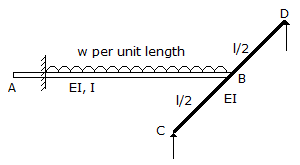# Civil Engineering - UPSC Civil Service Exam Questions

16.

The coordinates of two end-points A and B traverse line AB are XA = 1000.00 m, YA = 1000.00 m XB = 2000.00 m, YB = 1000.00 m The bearing of the line AB will be

 A. 0°0'00" B. 60°0'00" C. 90°0'00" D. 180°0'00"

Explanation:

No answer description available for this question. Let us discuss.

17.

Consider the following statements relating to compressible flow :
1. In a contracting conduit, sonic velocity is reached in the minimum cross-sectional area.
2. In a contracting conduit, supersonic velocities are reached only in the expanding section downstream from the minimum section.
3. An expansion shockwave is physically possible and can be exhibited.
4. In the Laval nozzle it is possible to obtain a velocity greater than the velocity of sound in the expanding section of nozzle.
Of these statements :

 A. 1, 2 and 3 are correct B. 1, 2 and 4 are correct C. 2, 3 and 4 are correct D. 1, 3 and 4 are correct

Explanation:

No answer description available for this question. Let us discuss.

18.

Consider the following factors :
1. Elevation
2. Temperature
4. Trip length.
For finding the runway length, which of these are taken into reckoning ?

 A. 1 and 3 B. 2 and 4 C. 1 and 2 D. 1, 2, 3 and 4

Explanation:

No answer description available for this question. Let us discuss.

19.

Which one of the following is the reaction of the cantilever at B as shown in the figure ?A. 3/8 wl B. 5/8 wl C. 6/17 wl D. 3/21 wl

Explanation:

No answer description available for this question. Let us discuss.

20.

If a road surface is adequately superelevated on horizontal curve, which one of the following is the proper distribution of pressure on the vehicle wheels ?

 A. Pressure on both outer and inner wheels is equal B. Pressure on inner wheels is more than the outer wheels C. Pressure on inner wheels is less than the outer wheels D. Pressure on front wheels is thrice the pressure on rear wheels